Win up to 100% scholarship on Aakash BYJU'S JEE/NEET courses with ABNAT Win up to 100% scholarship on Aakash BYJU'S JEE/NEET courses with ABNAT

# JEE Main 2021 March 17 – Shift 2 Physics Question Paper with Solutions

JEE Main 2021 Physics question paper for March 17, Shift 2 exam is provided here. We are offering accurate solutions which will further help students to perform well in the JEE Main exam. Students can have a glance at the question paper and get a better understanding of the question types, difficulty level, marking scheme. JEE Main 2021 Physics March 17, Shift 2 paper has been solved by our subject experts.

JEE Main 2021 March 17th Shift 2 Physics Question Paper

Section – A

Question 1. Two identical blocks A and B each of mass m resting on the smooth horizontal floor are connected by a light spring of natural length L and spring constant K. A third block C of mass m moving with a speed v along the line joining A and B collides elastically with A. The maximum compression in the spring is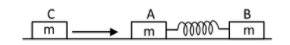a.

$$\begin{array}{l}\sqrt{\frac{mv}{2K}}\end{array}$$

b.
$$\begin{array}{l}\sqrt{\frac{m}{2K}}\end{array}$$

c.
$$\begin{array}{l}\sqrt{\frac{mv}{K}}\end{array}$$

d.
$$\begin{array}{l}v\sqrt{\frac{m}{2K}}\end{array}$$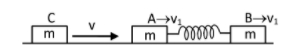Let v1 be the common velocity of blocks A and B at the maximum compression of the spring

From the conservation of momentum

mv = mv1 + mv1

v1 = V2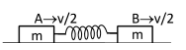& from energy conservation

$$\begin{array}{l}\frac{1}{2}mv^{2}= \frac{1}{2}m(\frac{v}{2})^{2}\times 2+\frac{1}{2}kx^{2}\end{array}$$
$$\begin{array}{l}\frac{mv^{2}}{2}-\frac{mv^{2}}{4}= \frac{1}{2}kx^{2}\end{array}$$
$$\begin{array}{l}\frac{mv^{2}}{4}= \frac{1}{2}kx^{2}\end{array}$$

The maximum compression in the spring is

$$\begin{array}{l}x = \sqrt{\frac{mv^{2}}{2K}}\end{array}$$
$$\begin{array}{l}x = v\sqrt{\frac{m}{2K}}\end{array}$$

Question 2. A solid sphere of mass 2 kg radius 0.5m is rolling with an initial speed of 1 ms-1 goes up an inclined plane which makes an angle of 300 with the horizontal plane, without slipping. How long will the sphere take to return to the starting point A?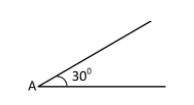a. 0.80 s
b. 0.60 s
c. 0.52 s
d. 0.57 s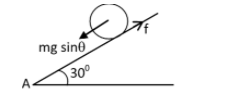$$\begin{array}{l}a = \frac{gsin\theta }{1+c}\end{array}$$

For a solid sphere, c = ⅖

$$\begin{array}{l}a = \frac{9.8 sin 30^{0} }{1+\frac{2}{5}}\end{array}$$

a = 3.5 m/sec2

Time of ascent is given by

v = u + at

0 = 1 – 3.5 t

$$\begin{array}{l}t= \frac{1}{3.5} sec\end{array}$$

Time of decent

$$\begin{array}{l}t= \frac{1}{3.5} sec\end{array}$$
(due to symmetry of motion)

Total time,

$$\begin{array}{l}T= \frac{2}{3.5} sec\end{array}$$
= 0.57 sec

Question 3. If one mole of a polyatomic gas has two vibrational modes and β is the ratio of molar specific heats for polyatomic gas β=Cp / Cv then the value of β is :

a. 1.35
b. 1.02
c. 1.25
d. 1.2

Degree of freedom of polyatomic gas

Here, no of translational dof = 3,

no of rotational dof = 3

and no of vibrational dof = 2×2 = 4

Therefore, total no of dof

f = 3 + 3 + 4 = 10

β = CP /CV =1 + (2/f) = 1+ (2/10)

β=12/10=1.2

Question 4. Two cells of emf 2E and E with internal resistance r1 and r2 respectively are connected in series to an external resistor R (see figure). The value of R, at which the potential difference across the terminals of the first cell becomes zero is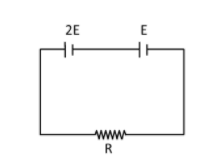a. r1 – r2
b. r1 + r2
c. (r1/2) + r2
d. (r1/2) – r2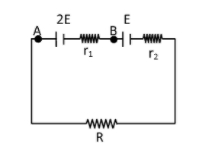$$\begin{array}{l}i = \frac{3E}{R+r_{1}+r_{2}}\end{array}$$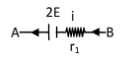If potential difference across terminals of first cell is zero

VA = VB

2 E = i r1

$$\begin{array}{l}2E = \frac{3E}{R+r_{1}+r_{2}}r_{1}\end{array}$$

2R + 2r1 + 2r2 = 3r1

R = r1/2- r2

Question 5. A sound wave of frequency 245 Hz travels with a speed of 300 ms-1 along the positive x-axis. Each point of the medium moves to and fro through a total distance of 6 cm. What will be the mathematical expression of the travelling wave?

a. Y (x,t) = 0.03 [ sin 5.1x – (0.2 x 103)t]
b. Y (x,t) = 0.06 [ sin 5.1x – (1.5 x 103)t]
c. Y (x,t) = 0.06 [ sin 0.8x – (0.5 x 103)t]
d. Y (x,t) = 0.03 [ sin 5.1x – (1.5 x 103)t]

General expression for wave travelling along positive x axis is of the form

Y = A sin (kx – ωt)

Here

A = 6/2 = 3cm = 0.03 m

ω = 2π f = 2π ×245

k = ω/v=(1.54×103)/300

k = 5.1 m-1

y = 0.03 sin (5.1x – 1.5 × 103t)

Question 6. A carrier signal C(t) = 25 sin (2.512×1010t) is amplitude modulated by a message signal m(t)= 5 sin (1.57×108t) and transmitted through an antenna. What will be the bandwidth of the modulated signal?

a. 1987.5 MHz
b. 2.01 GHz
c. 50 MHz
d. 8 GHz

Bandwidth of modulated signal is given by,

β = 2fm(t)

β = 2× {(1.57×108)/2π}

β = 50 MHz

Question 7. Two particles A and B of equal masses are suspended from two massless springs of spring constants K1 and K2 respectively. If the maximum velocities during oscillations are equal, the ratio of the amplitude of A and B is :

a.

$$\begin{array}{l}\frac{K_{1}}{K_{2}}\end{array}$$

b.
$$\begin{array}{l}\sqrt{\frac{K_{1}}{K_{2}}}\end{array}$$

c.
$$\begin{array}{l}\sqrt{\frac{K_{2}}{K_{1}}}\end{array}$$

d.
$$\begin{array}{l}\frac{K_{2}}{K_{1}}\end{array}$$

Since, Vmax = Aω

Given, A1ω1= A2ω2

We know that ω = √ k/m

$$\begin{array}{l}\sqrt{\frac{k_{1}}{m}}A_{1}= \sqrt{\frac{k_{2}}{m}}A_{2}\end{array}$$
$$\begin{array}{l}\frac{A_{1}}{A_{2}}=\sqrt{\frac{k_{2}}{k_{1}}}\end{array}$$

Question 8. Match List I with List II

 List I List II a) Phase difference between current and voltage in a purely resistive AC circuit i. 𝜋/2, current leads voltage b) Phase difference between current and voltage in a pure inductive AC circuit ii. zero c) Phase difference between current and voltage in a pure capacitive AC circuit iii. 𝜋/2, current lags voltage d) Phase difference between current and voltage in an LCR series circuit iv. tan-1 (Xc – XL/R)

Choose the most appropriate answer from the options given below :

a. (a)-(ii), (b)-(iii), (c)-(iv), (d)-(i)
b. (a)-(i), (b)-(iii), (c)-(iv), (d)-(ii)
c. (a)-(ii), (b)-(iv), (c)-(iii), (d)-(i)
d. (a)-(ii), (b)-(iii), (c)-(i), (d)-(iv)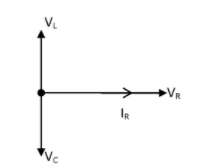(a) phase difference b/w current & voltage in a purely resistive AC circuit is zero

(b) phase difference b/w current & voltage in a pure inductive AC circuit is 𝜋/2; current lags voltage.

(c) phase difference b/w current & voltage in a pure capacitive AC circuit is 𝜋/2; current lead voltage.

(d) phase difference b/w current & voltage in an LCR series circuit is = tan-1 (Xc – XL/R)

Question 9. A geostationary satellite is orbiting around an arbitrary planet ‘P’ at a height of 11R above the surface of ‘P’, R being the radius of ‘P’. The time period of another satellite in hours at a height of 2R from the surface of ‘P’ is _____. ‘P’ has a time period of rotation of 24 hours.

a. 6/√ 2
b. 3
c. 6 √ 2
d. 5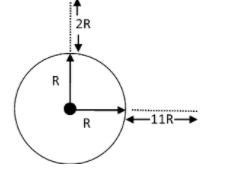From Kepler’s law

T2∝ R3

Since the time period of a geostationary satellite is equal to the time period of rotation of the planet i.e. 24 hours,

Using Kepler’s law,

(24/T)2= (12R/3R)3

T = 3 sec

Question 10. The velocity of a particle is v=v0+gt+Ft2. Its position is x=0 at t=0; then its displacement after time (t = 1) is :

a. v0+g/2+F
b. v0+2g+3F
c. v0+g+F
d. v0+g/2+F/3

v = v0 + gt + Ft2

dy/dt=v0+gt+Ft2

$$\begin{array}{l}\begin{array}{l} \int_{x=0}^{x} d x=\int_{t=0}^{t=1}\left(v_{0}+g t+F t^{2}\right) d t \\ x=\left[v_{o} t+\frac{g t^{2}}{2}+\frac{F t^{3}}{3}\right]_{t=0}^{t=1} \end{array}\end{array}$$

x=v0+g/2+F/3

Question 11. A block of mass 1 kg attached to a spring is made to oscillate with an initial amplitude of 12 cm. After 2 minutes the amplitude decreases to 6 cm. Determine the value of the damping constant for this motion. (take ln2 = 0.693).

a. 3.3×102 kg s-1
b. 5.7×10-3 kg s-1
c. 1.16 ×10-2 kg s-1
d. 0.69×102 kg s-1

Amplitude of a damped oscillation is given by;

$$\begin{array}{l}A = A_{0}e^{\frac{-b}{2m}t}\end{array}$$
$$\begin{array}{l}6 = 12e^{\frac{-b}{2\times 1}\times 120}\end{array}$$

6 = 12 e–b×60

1/2=e-60b

In(2) = 60b

b = ln(2)/60=1.16×10-2Kg/s

Question 12. An object is located 2 km beneath the surface of the water. If the fractional compression ∆V/V is 1.36%, the ratio of hydraulic stress to the corresponding hydraulic strain will be ……..

[Given: density of water is 1000 kgm–3 and g= 9.8 ms–2]

a. 2.26 ×109 Nm-2
b. 1.96 ×107 Nm-2
c. 1.44×107 Nm-2
d. 1.44×109 Nm-2

Bulk modulus

β = Δp/(ΔV/V)

$$\begin{array}{l}\beta =\frac{\rho gh}{\frac{\Delta V}{V}}=\frac{1000\times 9.8\times 2\times 10^{3}}{\frac{136}{100}}\end{array}$$

β = 1.44 × 109 N/m2

Question 13. Two identical photocathodes receive the light of frequencies f1 and f2 respectively. If the velocities of the photo-electrons coming out are v1 and v2 respectively, then

a.

$$\begin{array}{l}v_{1}+v_{2}=\left [ \frac{2h}{m}(f_{1}+f_{2}) \right ]^{1/2}\end{array}$$

b.
$$\begin{array}{l}v_{1}-v_{2}=\left [ \frac{2h}{m}(f_{1}-f_{2}) \right ]^{1/2}\end{array}$$

c.
$$\begin{array}{l}v_{1}^{2}+v_{2}^{2}=\left [ \frac{2h}{m}(f_{1}+f_{2}) \right ]\end{array}$$

d.
$$\begin{array}{l}v_{1}^{2}-v_{2}^{2}=\left [ \frac{2h}{m}(f_{1}-f_{2}) \right ]\end{array}$$

Using the expression for K.E of photoelectrons

$$\begin{array}{l}\frac{1}{2}mv_{1}^{2}=hf_{1}-\phi ———-(1)\end{array}$$
$$\begin{array}{l}\frac{1}{2}mv_{2}^{2}=hf_{2}-\phi ———-(2)\end{array}$$
[φ is the same for same material of photocathodes]

Subtracting equation (1) by equation (2)

$$\begin{array}{l}\frac{1}{2}mv_{1}^{2}-\frac{1}{2}mv_{2}^{2}=hf_{1}-hf_{2}\end{array}$$
$$\begin{array}{l}v_{1}^{2}-v_{2}^{2}=\frac{2h}{m}(f_{1}-f_{2})v_{1}^{2}-v_{2}^{2}=\frac{2h}{m}(f_{1}-f_{2})\end{array}$$

Question 14. The atomic hydrogen emits a line spectrum consisting of various series. Which series of hydrogen atomic spectra lie in the visible region?

a. Balmer series
b. Lyman series
c. Brackett series
d. Paschen series

Balmer series of hydrogen atomic spectrum is lying in the visible region when the electron jumps from a higher energy level to n = 2 orbit.

Question 15. Which one of the following will be the output of the given circuit?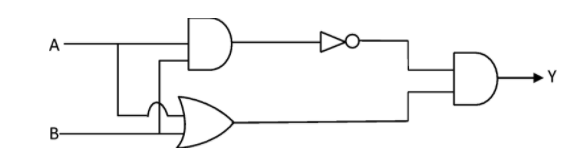a. NAND Gate
b. AND Gate
c. XOR Gate
d. NOR Gate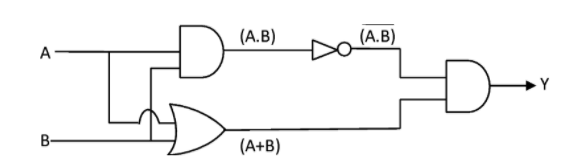Output of the above circuit can be obtained as

$$\begin{array}{l}\begin{array}{l} \mathrm{y}=(\underline{A \cdot B}) \cdot(A+B) \\ =\left(A^{-}+B^{-}\right) \cdot(A+B) \quad\\ =A^{-} A+A^{-} B+A B^{-}+B \\ =0+A^{-} B+A B^{-}+0 \\ \mathrm{y}=A^{-} B+A B^{-} \end{array}\end{array}$$

which is XOR gate

Question 16. The four arms of a Wheatstone bridge have resistances as shown in the figure. A galvanometer of 15 resistance is connected across BD. Calculate the current through the galvanometer when a potential difference of 10 V is maintained across AC.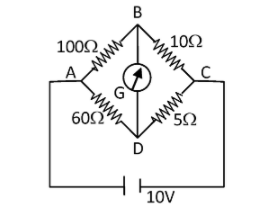a. 4.87 mA
b. 4.87 μA
c. 2.44 μA
d. 2.44 mA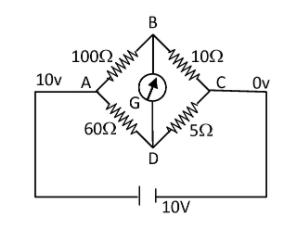Applying KCL for point B,

$$\begin{array}{l}\frac{V_{B}-10}{100}+\frac{V_{B}-V_{D}}{15}+\frac{V_{B}-0}{10}=0\end{array}$$
$$\begin{array}{l}\frac{V_{B}-10}{20}+\frac{V_{B}-V_{D}}{3}+\frac{V_{B}-0}{2}=0\end{array}$$

3VB-30+20VB-20VD+30VB=0

53VB – 20VD = 30 ____(1)

Similarly applying KCL for point D,

$$\begin{array}{l}\frac{V_{D}-10}{60}+\frac{V_{D}-V_{B}}{15}+\frac{V_{D}-0}{5}=0\end{array}$$

VD – 10 + 4VD – 4VB + 12VD = 0

– 4VB + 17VD = 10 _____(2)

after solving equation (1) & (2)

VD = 0.792 volt

VB = 0.865 volt

Then the current through the galvanometer

$$\begin{array}{l}=\frac{V_{B}-V_{D}}{R}\end{array}$$
$$\begin{array}{l}=\frac{0.865-0.792}{15}\end{array}$$

= 4.87 mA

Question 17. Which one is the correct option depicting the two different thermodynamic processes?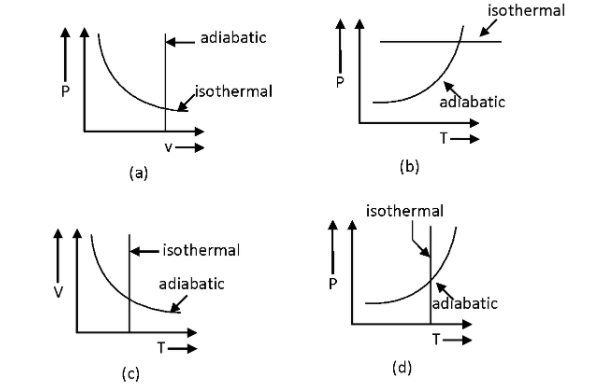a. (c) and (d)
b. (b) and (c)
c. (a) only
d. (c) and (a)

Isothermal process means constant temperature which is only possible in the graph (c) & (d)

PVγ = constant ——-(1)

Therefore, PV = nRT

P∝T/V

So, (T/V)Vγ = constant

TVγ-1 = constant ——-(2)

Similarly,

V ∝ T/P

P(T/P)γ = constant

P1- γTγ = constant ——-(3)

Therefore, differentiating equation (3) w.r.t temp

P1-γγTγ-1dT+Tγ(1-γ)P1-γ-1dP=0

$$\begin{array}{l}\frac{dP}{dT}=\left ( \frac{\gamma }{1-\gamma } \right )\left ( \frac{P}{T} \right )\end{array}$$
$$\begin{array}{l}\frac{dP}{dT}=\left ( \frac{\gamma }{\gamma-1 } \right )\left ( \frac{P}{T} \right )\end{array}$$

It gives (+ve) slope.

Question 18. A hairpin-like shape as shown in the figure is made by bending a long current-carrying wire. What is the magnitude of a magnetic field at point P which lies on the centre of the semicircle?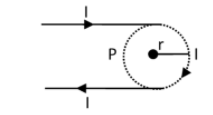a.

$$\begin{array}{l}\frac{\mu _{0}I}{4\pi r}(2-\pi )\end{array}$$

b.
$$\begin{array}{l}\frac{\mu _{0}I}{4\pi r}(2+\pi )\end{array}$$

c.
$$\begin{array}{l}\frac{\mu _{0}I}{2\pi r}(2+\pi )\end{array}$$

d.
$$\begin{array}{l}\frac{\mu _{0}I}{2\pi r}(2-\pi )\end{array}$$

Magnetic field due to each of the straight wire =

$$\begin{array}{l}\frac{\mu _{0}I}{4\pi r}\end{array}$$

Magnetic field due to semicircular arc =

$$\begin{array}{l}\frac{\mu _{0}I}{4r}\end{array}$$

Thus, the total magnetic field at point P

$$\begin{array}{l}B=\frac{\mu _{0}I}{4\pi r}+\frac{\mu _{0}I}{4\pi r}+\frac{\mu _{0}I}{4r}\end{array}$$
$$\begin{array}{l}B=\frac{\mu _{0}I}{2\pi r}+\frac{\mu _{0}I}{4r}\end{array}$$
$$\begin{array}{l}B=\frac{\mu _{0}I}{4\pi r}(2+ \pi )\end{array}$$

Question 19. A rubber ball is released from a height of 5 m above the floor. It bounces back repeatedly, always rising to (81/100) of the height through which it falls. Find the average speed of the ball. (Take g=10 ms-2)

a. 2.50 ms-1
b. 3.50 ms-1
c. 3.0 ms-1
d. 2.0 ms-1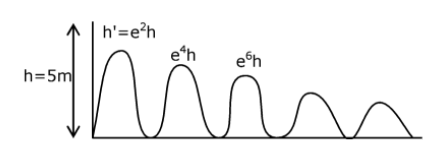Total distance d = h + 2e2h + 2e4h + 2e6h + 2e8h +….

d = h + 2e2h (1+e2+e4+e6+…..)

$$\begin{array}{l}d= h+2e^{2}h(\frac{1}{1-e^{2}})\end{array}$$
$$\begin{array}{l}d= \frac{(1-e^{2})h+2e^{2}h}{1-e^{2}}= \frac{h(1+e^{2})}{1-e^{2}}\end{array}$$

Total time = T + 2 eT + 2e2T + 2e3 T +…..

Total time = T + 2e T (1+e + e2 + e3+……)

$$\begin{array}{l}= T + 2e.T(\frac{1}{1-e})\end{array}$$

Total time =

$$\begin{array}{l}= \frac{T(1+e)}{1-e}\end{array}$$

Average speed of the ball

$$\begin{array}{l}\begin{array}{l} V_{\text {avg }}=\frac{h \frac{\left(1+\varepsilon^{2}\right)}{\left(1-s^{2}\right)}}{T\left(\frac{1+s}{1-\varepsilon}\right)} \\ =\frac{5}{1}\left(\frac{1+e^{2}}{(1+e)(1-e)} \frac{(1-e)}{(1+e)}\right) \\ V_{\text {avg }}=\frac{5\left(1+e^{2}\right)}{(1+e)^{2}} \end{array}\end{array}$$

Therefore, h’ = e2h

(81/100) = e2

e = 9/10 = 0.9

$$\begin{array}{l}V_{avg}= \frac{5(1+\frac{81}{100})}{(1+0.9)^{2}}\end{array}$$

= 2.50 m/sec

Question 20. What happens to the inductive reactance and the current in a purely inductive circuit if the frequency is halved?

a. Both, including reactance and current, will be doubled
b. Both, inductive reactance and current will be halved
c. Inductive reactance will be halved and current will be doubled
d. Inductive reactance will be doubled and current will be halved

XL = ωL

If the frequency is halved, X’L=(XL/2)

[inductive reactance is halved]

Therefore, I = V/XL

& I’ = 2V/XL=2I [current will be doubled]

SECTION –B

Question 21. The electric field intensity produced by the radiation coming from a 100 W bulb at a distance of 3 m is E. The electric field intensity produced by the radiation coming from 60 W at the same distance is √(x/5)E. Where the value of x = ________

I = 1/2C∈0E2

where E is electric field intensity at a point

E2 ∝ I

I = Power/Area

E2 ∝ (P/A)

E ∝ √P

[at the same distance, A will be the same]
$$\begin{array}{l}\frac{E’}{E}=\sqrt{\frac{60}{100}}\end{array}$$
$$\begin{array}{l}E’=\sqrt{\frac{3}{5}}E\end{array}$$

So the value of x = 3

Question 22. The image of an object placed in the air formed by a convex refracting surface is at a distance of 10 m behind the surface. The image is real and is at 2nd/3 of the distance of the object from the surface. The wavelength of light inside the surface is 2/3 times the wavelength in air, The radius of the curved surface is x/13 m. The value of ‘x’ is _____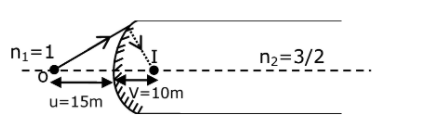$$\begin{array}{l}\frac{n_{2}}{v}-\frac{n_{1}}{u}=\left ( \frac{n_{2}-n_{1}}{R} \right )\end{array}$$

Since wavelength of light inside the surface is 32 times the wavelength in air,

$$\begin{array}{l}n_{2} = \frac{3}{2}\times n_{1}=\frac{3}{2}\end{array}$$
$$\begin{array}{l}\frac{\frac{3}{2}}{10}-\frac{1}{-15}=\frac{\frac{3}{2}-1}{R}\end{array}$$
$$\begin{array}{l}\frac{3}{20}+\frac{1}{15}=\frac{1}{2R}\end{array}$$

R =150/65)

R = (30/13)

Thus, the value of x = 30

Question 23. A 2μF capacitor C1 is first charged to a potential difference of 10V using a battery. Then the battery is removed and the capacitor is connected to an uncharged capacitor C2 of 8 μF. The charge in C2 on equilibrium condition is ________ μC. (Round off to the Nearest Integer)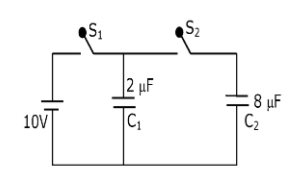After capacitor C1 is fully charged,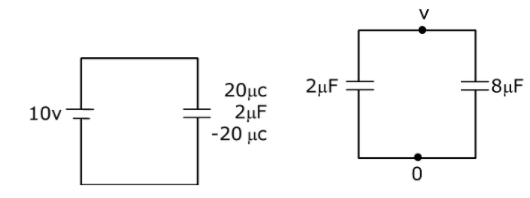When battery is removed & the capacitor is connected

At equilibrium condition, let voltage across each capacitor be V.

Then, using conservation of charge

2V + 8V = 20

10V = 20

V = 2 volt

Q = CV

Q = 8×2 = 16μc

Question 24. A particle of mass m moves in a circular orbit in a central potential field U(r) =U0r4. If Bohr’s quantization conditions are applied, radii of possible orbital rn vary with n1/α, where α is _____

$$\begin{array}{l}\vec{F}=-\frac{\vec{du}}{dr}\end{array}$$
$$\begin{array}{l}\vec{F}=-\frac{\vec{d}}{dr}(U_{0}r^{4})\end{array}$$
$$\begin{array}{l}\vec{F}=-4U_{0}r^{3}\end{array}$$

Therefore, mv2/r = 4U0r3

Then, v∝r2

Therefore, mvr = nh/2𝜋

Thus, r3α n

r α (n)1/3

So the value of α = 3

Question 25. The electric field in a region is given by

$$\begin{array}{l}\vec{E}=\frac{2}{5}E_{0}i+\frac{3}{5}E_{0}j\end{array}$$
with E0=4.0×103N/C. The flux of this field through a rectangular surface are 0.4 m2 parallel to Y–Z plane is ________Nm2C-1.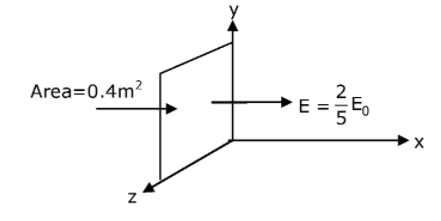From Gauss’ law

$$\begin{array}{l}\phi =\oint \vec{E}.d\vec{A}\end{array}$$

= (2/5) E0 x (0.4)

= (2/5) x 4 x 103 x 0.4

Φ = 640 Nm2 c-1

Question 26. A body of mass 1 kg rests on a horizontal floor with which it has a coefficient of static friction 1/√3. It is desired to make the body move by applying the minimum possible force F N. The value of F will be __________. (Round off to the Nearest Integer)

[Take g = 10 ms-2]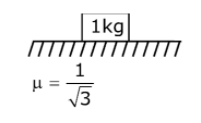Minimum possible force ⇒

$$\begin{array}{l}F = \frac{\mu mg}{\sqrt{1+\mu ^{2}}}\end{array}$$
$$\begin{array}{l}F_{mm} = \frac{\frac{1}{\sqrt{3}} \times 1\times 10}{\sqrt{1+\frac{1}{3}}}\end{array}$$

Fmin = 5 N

Question 27. Seawater at a frequency f = 9 × 102 Hz, has permittivity ∈ = 80∈0 and resistivity ρ = 0.25 Ωm. Imagine a parallel plate capacitor is immersed in seawater and is driven by an alternating voltage source V(t) = V0 sin(2πft). Then the conduction current density becomes 10x times the displacement current density after time t= 1800. The value of x is __________.

(Given : 1/4𝜋∈0 = 9 × 109Nm2C-2)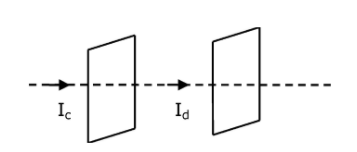Given:-

f = 9 × 102 Hz

∈=∈0r

∈=80∈0

So, ∈r=80

ρ = 0.25 Ωm

V(t) = V0 sin (2πft)

Displacement current, Id = dq/dt=(cdv/dt)

$$\begin{array}{l}I_{d}=\frac{\epsilon _{0}\epsilon _{r}A}{d}\frac{d}{dt}(v_{0}sin(2\pi ft))\end{array}$$
$$\begin{array}{l}I_{d}=\frac{\epsilon _{0}\epsilon _{r}A}{d}V_{0}(2\pi f)cos(2\pi ft)—–(1)\end{array}$$

Where d is the distance between plates & conduction current Ic = V/R

$$\begin{array}{l}I_{c}= \frac{V_{0}sin(2\pi ft)}{\rho \frac{d}{A}}=\frac{AV_{0}sin(2\pi ft)}{\rho d}——(2)\end{array}$$

Divide equation (1) and (2)

$$\begin{array}{l}\frac{I_{d}}{I_{c}}= \epsilon _{0}\epsilon _{r}2\pi f(\rho )cot(2\pi ft)\end{array}$$
$$\begin{array}{l}\frac{I_{d}}{I_{c}}= \frac{1}{4\pi \times 9\times 10^{9}}\times 80\times 2\pi \times 9\times 10^{2}\times (0.25)\times cot(2\pi \times9\times 10^{2}\times \frac{1}{800} )\end{array}$$
$$\begin{array}{l}= \frac{10^{3}}{10^{9}}(cot(\frac{9\pi }{4}))\end{array}$$
$$\begin{array}{l}= \frac{10^{3}}{10^{9}}\end{array}$$
$$\begin{array}{l}\frac{I_{d}}{I_{c}}=\frac{1}{10^{6}}\end{array}$$

Ic = 106 Id

So x = 6

Question 28. The disc of mass M with uniform surface mass density σ is shown in the figure. The centre of mass of the quarter disc (the shaded area) is at the position x/3, R/𝜋,xR/3𝜋. x is ____(Round off to the Nearest Integer) [a is an area as shown in the figure]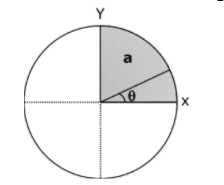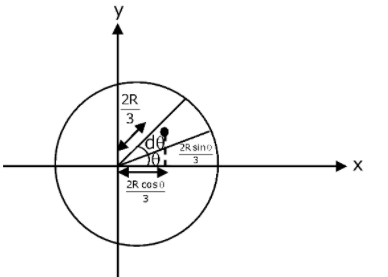Each segment of disc subtending angle dθ can be considered as a triangle of height R and base Rdθ

Mass of a segment of disc subtending angle dθ

d = σ (½) R ×Rdθ

d = (σR2dθ)/2

Therefore, x coordinate of COM of quarter disc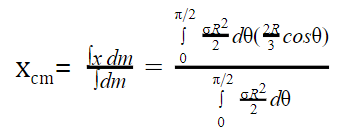Here, x is the x-coordinate of COM of dm mass. And for a triangular section it’s COM is at a cross section of median which will be at a distance 2R/3 from vertex. That’s why they have taken x-coordinate as (2R/3)cosθ

$$\begin{array}{l}=\frac{2 R}{3} \frac{\int_{0}^{\pi / 2} \cos \theta d \theta}{\int_{0}^{\pi / 2} d \theta}\end{array}$$

= (2R/3)(2/𝜋)

= 4R/3π

So the value of x = 4

Question 29. Suppose you have taken a dilute solution of oleic acid in such a way that its concentration becomes 0.01 cm3 of oleic acid per cm3 of the solution. Then you make a thin film of this solution (monomolecular thickness) of area 4 cm2 by considering 100 spherical drops of radius (3/4𝜋) x 10-3 cm. Then the thickness of the oleic acid layer will be x × 10-14 m where x is _________.

Volume of film = Area of film × thickness

Also, we know: Volume of film = Volume of 100 spherical drops

4tT=100×(4/3)πr3

=100×(4π/3) x (3/40π) x 10-9

=10-8 cm3

Hence, the thickness of the film;

tT=25×10-10cm

=25×10-12 m

& Thickness of oleic acid layer

t0=0.01

tT=25×10-14m

So, x = 25

Question 30. A boy of mass 4 kg is standing on a piece of wood having mass 5 kg. If the coefficient of friction between the wood and the floor is 0.5, the maximum force that the boy can exert on the rope so that the piece of wood does not move from its place is ________N. (Round off to the Nearest Integer)

[Take g = 10 ms-2]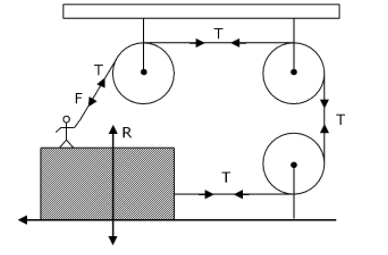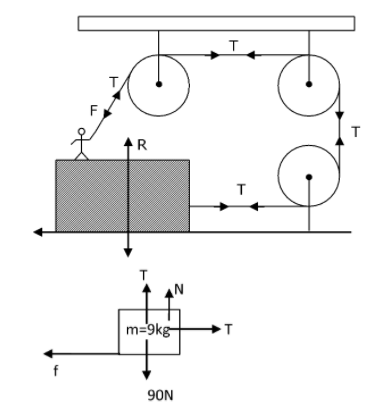[R is the reaction force between man and block while N from ground to block]

From FBD of [man + wooden block] system

Therefore, f=T [horizontal direction]

μN = T

μ(90-T) = T

[Because T + N = 90 (in vertical direction)]

0.5 (90–T) = T

90–T = 2T

3T = 90 T = 30 N